### 3D Geometry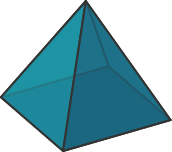How many of these could be an unfolded version of the pyramid shown above?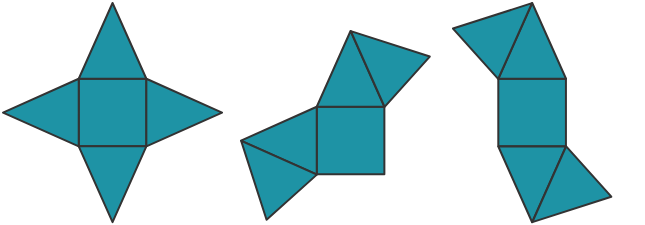# Surfaces of Shapes

Which of these shapes will successfully fold up to make a cube? (The cube must be closed with no overlapping faces.)# Surfaces of Shapes

When this shape is folded up into a cube, which edge color touches the red edge at the top of the figure?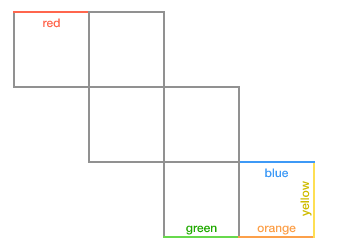# Surfaces of Shapes

So far, we've treated geometric solids in an abstract way, but now let's consider what happens when we add some real measurements.

How long is the orange path between the blue point and the red point on the surface of this $3 \times 3 \times 3\text{ m}$ cube?

# Surfaces of Shapes

By unfolding the cube from the last question, we can see how to solve for the length of the path.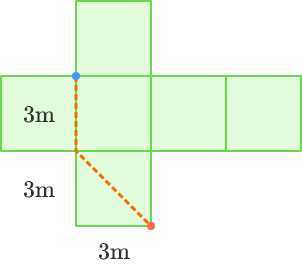From this view, we can also see that this path is not the shortest path between the two points.

# Surfaces of Shapes

Assuming we can travel on the outside of the cube but not through it, what is the length of the shortest path from the blue point on the $3 \times 3 \times 3\text{ m}$ cube to the red point on the opposite vertex of the cube?

# Surfaces of Shapes

Nets are a powerful tool for visualizing and understanding 3D shapes.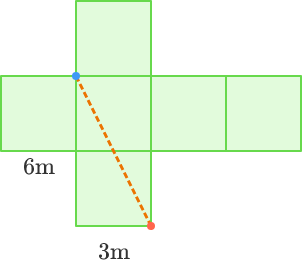In this course, we'll show you how to use nets to solve all kinds of different problems.

×